Physic Tutorials

# Notes on current of Electricity for Cambridge A level and UTME

Notes on current of Electricity for Cambridge A level and UTME

Current of Electricity

Electric charge Q passing a point is defined as the product of the (steady) current at that point and the time for which the current flows.

Q = It

Q = ne

I is the current

t is the time taken

When charges flow, there is electric current  I, therefore,

Electric current is the rate of flow of electric charge

I = ΔQ/Δt

The conventional direction of current is that of the +ve charge i.e current moves from the positive terminal to the negative terminalOne coulomb: is defined as the charge flowing per second past a point at which the current is one ampere.

Potential difference: Potential difference is defined as the energy transferred from electrical energy to other forms of energy e.g heat, light, sound e.t.c, when unit charge passes through an electrical device.

W = QV

W is the workdone

Q is the electric charge

v is the potential difference

V = W/ Q, the unit is JC-1 or volt

The volt: is defined as the potential difference between two pts in a circuit in which one joule of energy is converted from electrical to non-electrical energy when one coulomb passes from one point to the other, i.e 1 volt = 1 JC-1.

Resistance: is defined as the ratio of the potential difference across a component to the current flowing through it, provided temperature( and other physical conditions like resistivity, area of cross section, and length of wire remains constant.)

The Ohm: is the resistance of a resistor if there is a current of 1 A flowing through it when the p.d across it is 1 V, i.e,
1 Ω = One volt per ampere

R = V/I

Power is the rate of energy expended

P = E / t

E = Pt

V = W/Q

Substitute for E

V = Pt / Q

Q = It

t = Q / I

Substitute for t

V = PQ/IQ

V = P /I

P = IV ……….i

Note

V = IR

substitute for V

P = I^2 R

sketch and explain the I-V characteristics of a metallic conductor at constant temperature, a semiconductor diode and a filament lamp

For Metallic conductor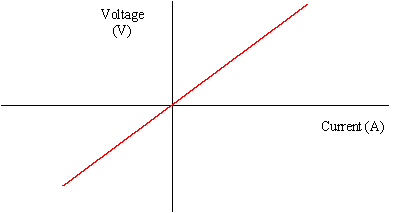For filament lamp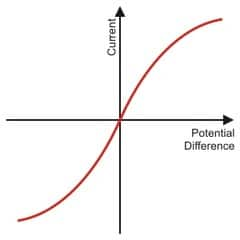For semiconductor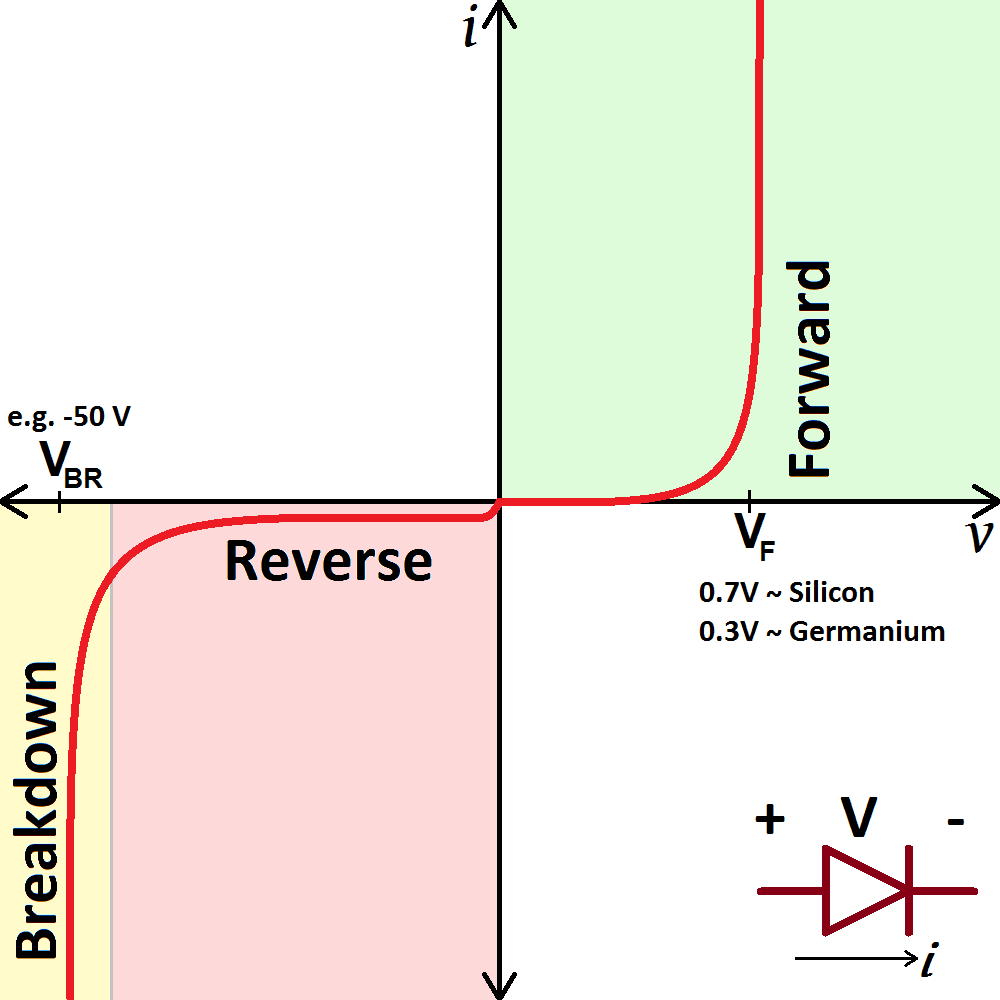Ohm’s Law:  The current flowing through a piece of metal is proportional to the potential difference across it providing the temperature remains constant

From the ohm’ law equation for ohmic conductors,

(1) as R increases, the p.d drawn by the load increases, and vice versa, but R is inversely proportional to I, so current decreases because, as resistance increases, current decreases and vice versa.

(2)for a resistor and/(variable) resistor, as the R of one decreases, the p.d of the other increases, its current also increases, because, the

(3)V/I is always a constant value at the same temperature

Resistivity

Resistivity is defined as the resistance of a material of unit cross-sectional area and unit length.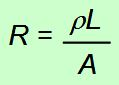R = Resistance

r = Resistivity of material

L = Length of conductor

A = Area

E.m.f and P.d

Electromotive force Ɛ is defined as the(total) energy transferred / converted from non-electrical forms into electrical energy when unit charge is moved round a complete circuit.

distinguish between e.m.f. and p.d. in terms of energy considerations

e.m.f.  = (energy converted from other forms to electrical) / charge

p.d. = (energy converted from electrical to other forms) / charge

Internal resistance

Internal resistance is the resistance to current flow within the power source. It reduces the potential difference (not  the emf) across the terminal of the power supply when it is delivering a current.

Consider the circuit below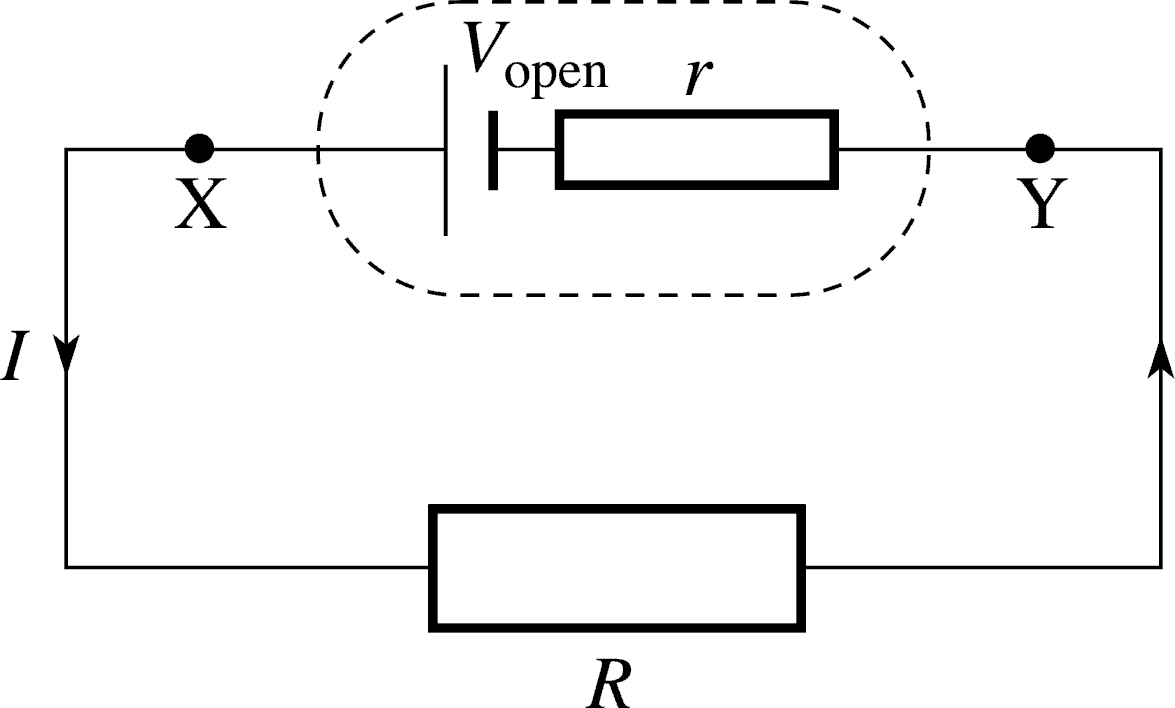E.m.f = I(R +r)

E.m.f = IR +Ir

IR is the terminal P.d

Ir is the lost volt

The greater the internal resistance, then the greater percentage loss in energy per unit charge, and the lower the terminal p.d V( = IR), and vice versa. So reducing the internal resistance of a battery increases the effective/useful energy delivered per unit charge across the external load.